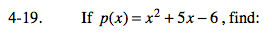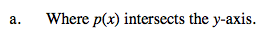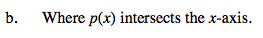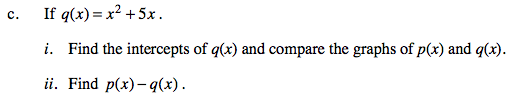### Home > A2C > Chapter 4 > Lesson 4.1.2 > Problem4-19

4-19.Set x to 0: p(x) = (0)2 + 5(0) − 6

p(x) intersects the y-axis at (0, −6).Set y to 0: (0) = x2 + 5x − 6

0 = (x + 6)(x − 1)

p(x) intersects the x-axis at (−6, 0) and (1, 0).Follow the steps in parts (a) and (b).

How are the positions of the graphs different? Are their sizes different?

Subtract the two expressions.